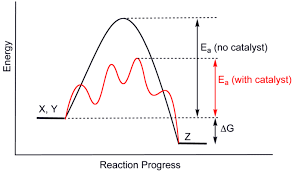## How to Calculate and Solve for Rate of Heterogeneous Reaction | CorrosionThe image above represents rate of heterogeneous reaction.

To compute for rate of heterogeneous reaction, two essential parameters are needed and these parameters are dn/dt and Amount of Material (n).

The formula for calculating rate of heterogeneous reaction:

V = dn/dt / n

Where:

V = Rate of Heterogeneous Reaction
dn/dt = dn/dt
n = Amount of Material

Let’s solve an example;
Find the rate of heterogeneous reaction when the dn/dt is 36 and the amount of material is 12.

This implies that;

dn/dt = dn/dt = 36
n = Amount of Material = 12

V = dn/dt / n
V = 24 / 12
V = 2

Therefore, the rate of heterogeneous reaction is 2.

Calculating the dn/dt when the Rate of Heterogeneous Reaction and the Amount of Material is Given.

dn/dt = V x n

Where;

dn/dt = dn/dt
V = Rate of Heterogeneous Reaction
n = Amount of Material

Let’s solve an example;
Find the dn/dt when the rate of heterogeneous reaction is 28 and the amount of material is 2.

This implies that;

V = Rate of Heterogeneous Reaction = 28
n = Amount of Material = 2

dn/dt = V x n
dn/dt = 28 x 2
dn/dt = 56

Therefore, the dn/dt is 56.